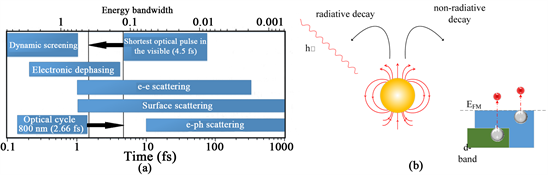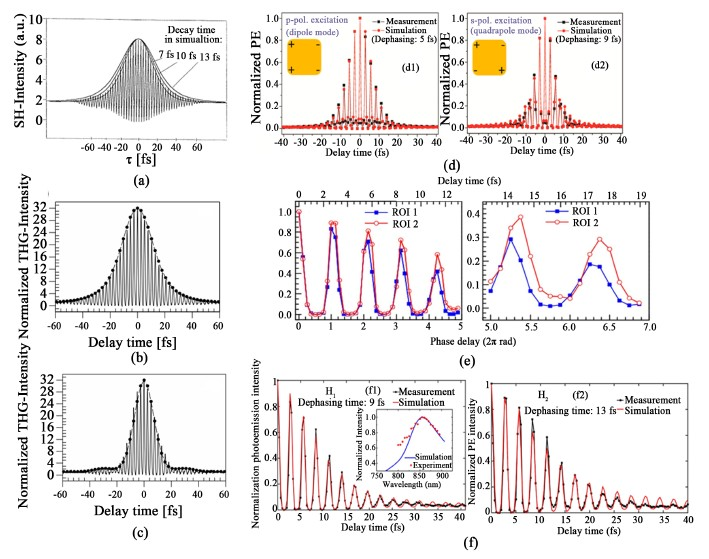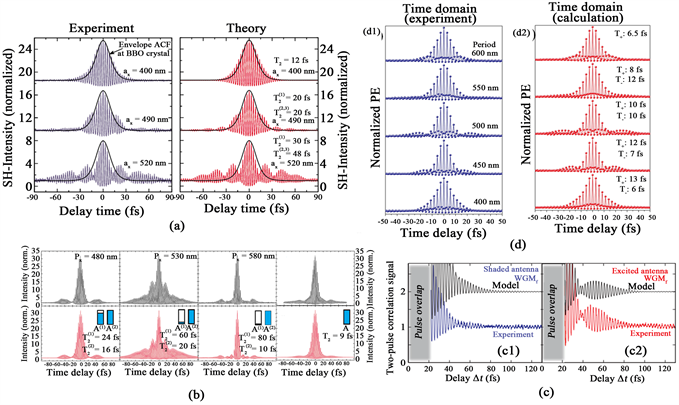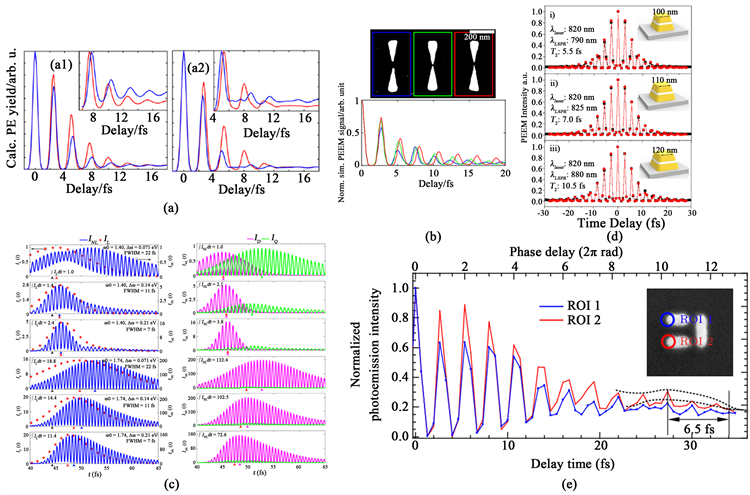# 利用PEEM对等离激元场动力学演化的高时空分辨近场表征研究进展 Research Progress in High Spatial-Temporal Near-Field Characterization of Dynamical Evolution of Plasmon Fields Using PEEM

• 全文下载: PDF(4535KB)    PP.274-286   DOI: 10.12677/APP.2019.95033
• 下载量: 278  浏览量: 407

Plasmon is widely used in research fields such as sensing, photovoltaic, photocatalysis and photodetection for its characteristics of super-diffraction limit convergence and high near-field enhancement. Ultrafast plasmon field formed by ultrafast light-irradiated metal nanostructures not only has traditional plasmon characteristics, but also has very small time-scale characteristics, which provides an effective method for searching and manipulating the interaction between light and matter at femtosecond and nanoscale. Since the dynamical evolution process of the plasmon field directly affects the performance of plasmon sensing and detecting devices as well as the photocatalytic effect, directly near-field characterization of the dynamic evolution of the plasmon field will help the exploration and revealing of the interaction between plasmon and
matter in microscopic scale, which is of great significance for the design and optimization of plasmonic nano-photoelectric devices. This paper reviews the advances in high spatial revolution near-field characterization of dynamical evolution of plasmon fields using ITR-PEEM.

1. 引言

Heilweil和Hochstrasser论证了均匀线宽和去相位时间之间存在如下关系  ：

$\nu =\frac{h}{2\text{π}{T}_{2}}$ (1)

2. 等离激元动力学理论

2.1. 等离激元的动力学过程Figure 1. (a) Overall dephasing process of SPPs  ; (b) Radiative and non-radiative damping of LSPs 

$\frac{1}{{T}_{2}}=\frac{1}{2T}+\frac{1}{{T}_{2}^{*}}$ (2)

2.2. 超快等离激元动力学演化计算理论模型

2.2.1. 简单谐振子模型

B. Lamprecht课题组在1999年证实了阻尼谐振子模型可用于表征超快LSPs的动力学特性  ，其中，体等离激元场遵循以下公式  ：

${E}_{pl}\left(t\right)\propto {\int }_{-\infty }^{t}K\left({t}^{*}\right){\text{e}}^{-\gamma \left(t-{t}^{*}\right)}\mathrm{sin}\left[{\omega }_{0}\left(t-{t}^{*}\right)\right]\text{d}{t}^{*}$ (3)

${I}^{\left(3\omega \right)}\propto \int {|{E}_{pl}\left(t\right)|}^{6}\text{d}t$ (4)

2.2.2. 多模式耦合谐振子模型

${\partial }_{t}^{2}{E}_{1}^{loc}\left(t\right)+2{\gamma }_{1}{\partial }_{t}{E}_{1}^{loc}\left(t\right)+{\omega }_{1}^{2}{E}_{1}^{loc}\left(t\right)+\kappa {E}_{2}^{loc}\left(t\right)={E}_{laser}\left(t\right)$ (5)

${\partial }_{t}^{2}{E}_{2}^{loc}\left(t\right)+2{\gamma }_{2}{\partial }_{t}{E}_{2}^{loc}\left(t\right)+{\omega }_{2}^{2}{E}_{2}^{loc}\left(t\right)+\kappa {E}_{1}^{loc}\left(t\right)=0$ (6)

${R}_{1}\left(\omega \right)=\frac{-\left({\omega }_{2}^{2}+2{\gamma }_{2}i\omega -{\omega }^{2}\right)}{{\kappa }^{2}-\left({\omega }_{2}^{2}+2{\gamma }_{2}i\omega -{\omega }^{2}\right)\left({\omega }_{1}^{2}+2{\gamma }_{1}i\omega -{\omega }^{2}\right)}$ (7)

${R}_{2}\left(\omega \right)=\frac{\kappa }{{\kappa }^{2}-\left({\omega }_{2}^{2}+2{\gamma }_{2}i\omega -{\omega }^{2}\right)\left({\omega }_{1}^{2}+2{\gamma }_{1}i\omega -{\omega }^{2}\right)}$ (8)

$R\left(\omega \right)={a}_{1}{R}_{1}\left(\omega \right)+{a}_{2}{R}_{2}\left(\omega \right)$ (9)

$\begin{array}{c}{E}_{loc}\left(t,\tau \right)={E}_{laser}\left(t\right)\ast R\left(t\right)=\left(E\left(t\right)+E\left(t-\tau \right)\right)\ast R\left(t\right)\\ ={\int }_{-\infty }^{+\infty }E\left(\omega \right)R\left(\omega \right)\left(1+{\text{e}}^{-i\omega \tau }\right){\text{e}}^{i\omega t}\text{d}\omega \end{array}$ (10)

$S\left(\tau \right)={\int }_{-\infty }^{\infty }{|{E}_{loc}\left(t,\tau \right)|}^{2n}\text{d}t$ (11)

$F\left(\tau \right)=\underset{k}{\overset{N}{\sum }}{|S{\left({\tau }_{k}\right)}_{Exp}-S\left({\tau }_{k}\right)|}^{2}$ (12)

${\tau }_{k}={\tau }_{\mathrm{min}}+k\Delta \tau$ (13)

2.2.3. 半解析模型

Fraggiani课题组提出了一种基于时域共振模式扩展理论的半解析模型来处理光学纳米天线的时域动力学问题 。首先他们假设入射光场为 ${E}_{inc}\left(r,t\right)$ ，经过傅里叶变换可将其从时域变换到频率，得到 ${E}_{inc}\left(r,\omega \right)$ ，受到入射场的作用，纳米结构会产生散射发出电磁波 ${E}_{sca}\left(r,t\right)$ ，再经过傅里叶变换，并且每个入射场的无穷小频段的入射场可表示为 ${E}_{inc}\left(r,\omega \right)\text{d}\omega$ ，对应散射则为 ${E}_{sca}\left(r,\omega \right)\text{d}\omega$ ，将其做准正态模式(quasi-normal-mode (QNM))展开，得到：

$\text{d}{E}_{sca}\left(r,\omega \right)\approx {\sum }_{m}\text{d}{\beta }_{m}\left(\omega \right){E}_{m}\left(r\right)$ (14)

$\text{d}{\beta }_{m}\left(\omega \right)=\frac{-\omega \text{d}\omega }{\omega -{\omega }_{m}}\iiint \Delta \epsilon \left(r,\omega \right){E}_{inc}\left(r,\omega \right)\cdot {E}_{m}\left(r\right)\text{d}{r}^{3}$ (15)

${E}_{sca}\left(r,t\right)\approx \underset{m}{\sum }{\beta }_{m}\left(t\right){E}_{m}\left(r\right)$ (16)

$\begin{array}{c}{\beta }_{m}\left(t\right)={\int }_{-\infty }^{+\infty }\text{d}{\beta }_{m}\left(\omega \right)\mathrm{exp}\left(i\omega t\right)\\ ={\int }_{-\infty }^{+\infty }\frac{\omega \mathrm{exp}\left(i\omega t\right)}{{\omega }_{m}-\omega }\iiint \Delta \epsilon \left(r,\omega \right){E}_{inc}\left(r,\omega \right)\cdot {E}_{m}\left(r\right)\text{d}{r}^{3}\text{d}\omega \end{array}$ (17)

$E\left(r,t\right)={E}_{sca}\left(r,t\right)+{E}_{inc}\left(r,t\right)$ (18)

3. 等离激元动力学研究进展

3.1. 非耦合等离激元动力学研究

1999年B.Lamprecht用非谐振子模型，遵从等离子体振荡原理，对飞秒激光照射纳米结构的动力学响应进行了理论计算，并将其与实验所得的二次谐波自相关曲线进行拟合，得到了所选纳米结构的去相位时间 。如图2(a)所示，其展示的是分别用假定去相位时间为7 fs、10 fs、13 fs计算所得曲线和实验结果进行拟合，可知其去相位时间约为10 fs。但是，此方法受限于所选择的纳米结构必须为非中心对称结构  ，因此同年，其课题组借助三次谐波–自相关(THG-ACF)方法，采用理论和实验结合的方式研究了金纳米柱阵列激发的等离激元在共振和非共振情况下的动力学演化，并得到了等离激元的去相位时间，该模型的适用范围得到进一步推广 。如图2(b)，图2(c)所示，分别展示了在共振和非共振情况下，THG-ACF理论计算结果和实验所得自相关函数的拟合结果。这一研究也成为了后续利用ITR-PEEM技术进行等离激元动力学演化研究的基础 。Figure 2. Plasmon dynamics under non-coupling condition. (a) fitting between the analytical model and the ACF measured from the non-centrosymmetric silver nanostructures irradiated by femtosecond laser pulse  ; (b) fitting (resonant condition) between the analytical model and the ACF measured from the Au nanocylinder arrays irradiated by femtosecond laser pulse  ; (c) fitting (non-resonant condition) between the analytical model and the ACF measured from the Au nanocylinder arrays irradiated by femtosecond laser pulse  ; (d1) fitting between the analytical model and the ACF and the charge distribution on the Au nanoblock irradiated by p-polarized light  ; (d2) fitting between the analytical model and the ACF and the charge distribution on the Au nanoblock irradiated by s-polarized light  ; (e1) evolution of measured and simulated normalized photoemission signal from H1 spot  ; (e2) evolution of measured and simulated normalized photoemission signal from H2 spot 

3.2. 耦合等离激元动力学研究

3.2.1. LSP和SPP耦合Figure 3. Plasmon dynamics under LSP-SPP coupling condition. (a) measured second-order interferometric autocorrelation functions for different grating periods of the nanoparticle arrays and fitted ACFs with the obtained dephasing time  ; (b) measured (black cure) and calculated (red curve) nonlinear ACFs of different arrays on the waveguide;  (c1) correlation photoemission signals for shaded antennas  ; (c2) correlation photoemission signals for excited antennas  ; (d1) normalized PE intensity curves against the time delay in the time domain obtained by PEEM measurements  ; (d2) calculated PE signals with the best fitted dephasing time 

3.2.2. LSP和LSP耦合

Bernasconi研究组针对简单银纳米棒结构，利用二次谐波和全波数值方法，对飞秒激光照射该结构激发的等离激元的远场时域动力学展开研究。他们首先研究了线性响应，用以建立方法，发现观察到行为类似阻尼谐振子的单个本征模是有可能的。然后，他们研究了非线性散射场，首先，他们研究了纵四极模式在非线性频率下共振的情况，发现纵四极模式和横偶极模式在激发脉冲依然存在时发生干涉。最终，他们通过详细的模式分析，他们发现非线性辐射的多极特性受到脉冲的中心频率和宽度的极大影响，为线性和非线性LSP动力学的研究提供了新思路：通过改变入射光参数来实现不同的LSP动力学响应。如图4(c)所示，该图展示的是对线性和非线性辐射的多极分析 。Ueno研究组设计了一种金-氧化铝-金结构，利用上层和下层金结构产生的等离激元之间的耦合激发了等离激元暗模式，实现了3倍于金纳米块结构的去相位时间，并且通过改变多层结构的大小，证明了近场增强的大小取决于去相位时间的长短，随着去谐程度的减小，等离激元的去相位时间延长，就会造成更强的近场增强,并发现随着结构尺寸的增加，其去相位时间增加  ，如图4(d)所示。Lin课题组针对三个金纳米棒构成的石门结构，利用7 fs激光脉冲结合PEEM对该结构激发的超快等离激元进行了直接成像，并且他们发现由于宽谱超快激光脉冲的激发，在结构的末端出现了多个等离激元模式，在光辐射信号曲线上表现为拍频现象，如图4(e)所示。并且，通过控制泵浦光和探测光之间的延时，他们实现了对在该复杂系统的激发热点的控制，即实现了对等离激元动力学的控制 。Figure 4. Plasmon dynamics under LSP-LSP coupling condition. (a1) normalized near field autocorrelation trace at the two ends of nanorice with 500 nm length  ; (a2) normalized near field autocorrelation trace at the two ends of nanorice with 600 nm length  ; (b) SEM image of bowties with similar shape but slightly different lateral shape and corresponding autocorrelation traces  ; (c) multipolar analysis of the linear and nonlinear radiations. The red symbol and blue curve on the left part refer to linear and nonlinear scattered intensity respectively, and the pink and green curve on the right part refer to dipolar and quadrupolar components of the nonlinear scattered intensity  ; (d) the PEEM correlation curve (the black line) and the numerical simulations of the time-resolved nonlinear photoemission signal (the red line)  ; (e) evolution of the photoemission signals against delay time obtained from two ends of the dolmen 

4. 结束语

NOTES

*通讯作者。